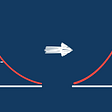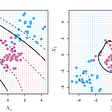# Sentiment Analysis on Alexa Reviews

Alexa is an AI-based virtual assistant developed by Amazon. It is today used in devices like Echo, Dot, and Firestick. It is capable of voice interaction, music playback, setting alarms, home automation, and providing weather information. We will do sentiment analysis on the reviews of Alexa products posted on Amazon. For this, we’ll use the Alexa Reviews dataset from Kaggle.

# Importing Libraries

Let’s first import the required libraries. If you don’t have a particular library installed, run the command ‘pip install <package_name>’ to install it.

`import osimport refrom string import punctuationfrom textblob import Wordimport pandas as pdimport matplotlib.pyplot as pltimport seaborn as snsfrom wordcloud import WordCloudimport nltkfrom nltk import word_tokenizefrom nltk.corpus import stopwordsfrom sklearn.model_selection import train_test_splitfrom sklearn.pipeline import Pipelinefrom sklearn.tree import DecisionTreeClassifierfrom sklearn.feature_extraction.text import CountVectorizer`

`nltk.download('punkt')nltk.download('stopwords')nltk.download('wordnet')`

We’ll have downloaded the dataset from Kaggle. Let us load it now. Note that the dataset is in a tab-separated format.

`data = pd.read_csv('./amazon_alexa_reviews/amazon_alexa.tsv',sep='\t')data.head()`

We will replace the column name ‘verified_reviews’ with ‘reviews’ for easy understanding.

`data.columns = data.columns.str.replace('verified_reviews', 'reviews')`

# Exploration & Preprocessing

Let’s see how many null values are present across different columns. The output shows that there are no null values in any column.

`data.isna().sum()`

We don’t need ‘date’ and ‘variation’ columns. So, we’ll delete them.

`data.drop(['date','variation'],axis=1,inplace=True)data.head()`

The rating 3 is neither positive nor negative. It is neutral, so we’ll remove it.

`data = data[data.rating != 3]`

Let us plot the distribution of rating values. The plot below shows that the rating of 5 has the highest occurrence, followed by 4, 1, and 2.

`#Using a countplotsns.set_palette(sns.color_palette('Set2'))sns.countplot(x='rating',data=data,order=data.rating.value_counts().index)plt.xlabel("rating")plt.title("Rating Distribution");`

We want to have only positive and negative target values. For this purpose, we will map 1,2 to 0 (negative), and map 4,5 to 1 (positive).

`#Replacing scores of 1,2 with 0 (negative) and 4,5 with 1 (positive)def score_sentiment(score):    if(score == 1 or score == 2):        return 0    else:        return 1data.rating = data.rating.apply(score_sentiment)`

Let’s plot the distribution of rating values after mapping.

`#Using a countplotsns.set_palette(sns.color_palette('Set2'))sns.countplot(x='rating',data=data,order=data.rating.value_counts().index)plt.xlabel("rating")plt.title("Rating Distribution");`

We will plot a pie chart to see the relative distribution of ‘feedback’ values. Feedback 1 means that the customer was satisfied and feedback 0 means that the customer wasn’t satisfied.

`sns.set_palette(sns.color_palette("hls", 8))data.feedback.value_counts().plot.pie()`

For the sentiment analysis, we’ll not need the ‘feedback’ column, so let’s delete it.

`data.drop('feedback',axis=1,inplace=True)`

We will create a function named clean to take care of the text preprocessing. The function clean will carry out the removal of HTML tags, punctuation, numbers, stop words, frequent characters. It will also carry out tokenization, case conversion, and lemmatization.

`def clean(dataframe):    #HTML tag removal    dataframe['reviews'] = dataframe['reviews'].apply(lambda words: re.sub('<.*?>','',words))    #Tokenization    dataframe['reviews'] = dataframe['reviews'].apply(word_tokenize)    #Upper to lower case    dataframe['reviews'] = dataframe['reviews'].apply(lambda words: [x.lower() for x in words])    #Punctuation removal    dataframe['reviews'] = dataframe['reviews'].apply(lambda words: [x for x in words if not x in punctuation])    #Number removal    dataframe['reviews'] = dataframe['reviews'].apply(lambda words: [x for x in words if not x.isdigit()])    #Stop word removal    dataframe['reviews'] = dataframe['reviews'].apply(lambda words: [x for x in words if x not in stopwords.words('english')])    #Frequent characters deleting    temp = dataframe['reviews'].apply(lambda words: " ".join(words))    freq = pd.Series(temp).value_counts()[:10]    dataframe['reviews'] = dataframe['reviews'].apply(lambda words: [x for x in words if x not in freq.keys()])    #Lemmatization    dataframe['reviews'] = dataframe['reviews'].apply(lambda words: " ".join([Word(x).lemmatize() for x in words]))    return dataframe`

Now, let’s apply the function clean to our dataframe and print its head.

`data = clean(datadata.head()`

Word Clouds are visual representations of words that appear more frequently in a text corpus. We will create word clouds for both positive and negative Alexa reviews, separately.

`#Function for creating a word clouddef wordcloud_draw(data, colormap):    words = ' '.join(data)    wordcloud = WordCloud(stopwords=stopwords.words('english')+  ['amazon','alexa','echo','dot','device'],    colormap=colormap,    width=2500,    height=2000    ).generate(words)    plt.figure(1,figsize=(10, 7))    plt.imshow(wordcloud)    plt.axis('off')    plt.show()#Segregating positive & negative reviewspositivedata = data[data['rating'] == 1]positivedata =positivedata['reviews']negdata = data[data['rating'] == 0]negdata= negdata['reviews']#Printing the word cloudsprint("For Positive reviews")wordcloud_draw(positivedata,colormap='Wistia')print("For Negative reviews")wordcloud_draw(negdata,colormap='tab20c')`

# Splitting the Data

We will split the data for training and testing purposes.

`X = data['reviews']Y = data['rating']x_train, x_test, y_train, y_test = train_test_split(X, Y, test_size=0.3)`

# Creating the Model

Let’s create a Pipeline now. A pipeline is used to sequentially apply a list of transforms and a final estimator. Our pipeline will contain CountVectorizer and DecisionTreeClassifier (the classifier we are going to use).

CountVectorizer will build a vocabulary of known words and encode new reviews using that vocabulary. The DecisionTreeClassifier will be used to classify the reviews into different classes. We will now fit the data using this pipeline.

`clf = Pipeline(steps =[('preprocessing', CountVectorizer()),('classifier', DecisionTreeClassifier(class_weight='balanced'))])clf.fit(x_train,y_train)`

We’ll calculate the score of our model. This will be done by checking the model’s performance on the test data. As shown in the output, the score is 0.893, which means our model classifies correctly 89.3% of the time.

`clf.score(x_test,y_test)`

# Prediction

It’s time to make a prediction. So, let’s predict the target class for the test data. The output shows the prediction array containing 0’s and 1’s.

The 0’s signify the classification into negative class and the 1’s signify the classification into positive class.

`clf.predict(x_test)`

We are done with the sentiment analysis of Alexa reviews. If you liked this story, do leave a clap!

--

--

--

## More from hackerdawn

hackerdawn is a place which you find stories that help you build stuff you’ve always wanted to. At hackerdawn, we always try to keep things simple and not bring complexity where it is not required.

## How Spotify’s shuffle algorithm works.## Power BI vs Tableau vs Knowi## ‘D&B Finance Analytics’ arrives to optimize the management of…## Use The German Concept of “Sehnsucht” to Help Chase What You Long For## What is Feature Scaling?## Bayesian Neural Networks: 2 Fully Connected in TensorFlow and Pytorch## Towards Data Analytics: Data literacy in R## Servian: Data Engineer Interview Questions## Examples of Supervised and Unsupervised Learning Methods for Sentiment Classification of Twitter…## Starbucks best suited offers based on customer demographics## Predicting YouTube Dislikes using Machine Learning